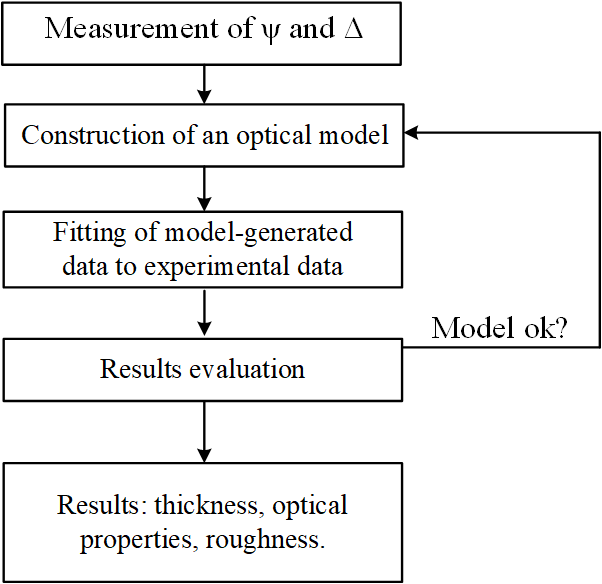# Spectroscopic ellipsometry

Spectroscopic ellipsometry (SE) is an optical technique which measures the change in polarisation when a light beam reflects from a sample as schematically shown in Figure 1. The change in the polarisation of light after reflection can be expressed by:$\frac{r_{p}}{r_{s}}=tan(\psi)e^{i\triangle}$(1)Figure 1: Schematic illustration of the working principle of spectroscopic ellipsometry. Adapted from .Figure 2: Main components of a typical spectroscopic ellipsometer. Adapted from .

A typical ellipsometer consists of a light source, polariser, sample stage, analyser, and detector as shown schematically in Figure 2. After obtaining the Ψ and Δ of the sample, this data can directly be converted into a so-called pseudo-dielectric function which can be useful in some cases. However, in most cases, the spectroscopic ellipsometry measurement is reproduced using computer software such as CompleteEase using an appropriate model of the sample. The model of the sample typically consists of a substrate with known optical properties and a thin film with known/unknown optical properties and thickness. The fitting procedure is schematically shown in Figure 3 and basically is an iterative approach where the mismatch between the measured and simulated ellipsometry measurement is minimised. In order to improve the measurement quality, often a sample is measured at various angles of incidence which than can be fitted simultaneously. This is particularly relevant for multilayer samples. When the optical properties of the thin film are not known oscillator models are used such as the Cauchy model or a Tauc-Lorentz oscillator which are appropriate for the type of material investigated. The optical function of a material can reveal a lot of information about the thin film of interest e.g. its composition, bandgap, crystallinity, active dopant concentration, etc.Figure 3: General process flow for the analysis of spectroscopic ellipsometry measurements. Adapted from .

A short instructive animation about spectroscopic ellipsometry is shown below.

References: